1. /
2. CBSE
3. /
4. Class 12
5. /
6. Computer Science
7. /
8. CBSE Question Paper 2010...

# CBSE Question Paper 2010 class 12 Computer Science### myCBSEguide App

Download the app to get CBSE Sample Papers 2023-24, NCERT Solutions (Revised), Most Important Questions, Previous Year Question Bank, Mock Tests, and Detailed Notes.

CBSE Question Paper 2010 class 12 Computer Science conducted by Central Board of Secondary Education, New Delhi in the month of March 2010. CBSE previous year question papers with solution are available in myCBSEguide mobile app and cbse guide website. The Best CBSE App for students and teachers is myCBSEguide which provides complete study material and practice papers to cbse schools in India and abroad.

CBSE Question Paper 2010 class 12 Computer Science

## Class 12 Computer Science list of chapters

1. Review of Python
2. Concept of Object Oriented Programming
3. Classes in Python
4. Inheritance
5. Linear List Manipulation
6. Stacks & Queues in list
7. Data File Handling
8. Exception Handling & Green Functions
9. Databases Concepts and SQL
10. Structure  Query Language
11. Boolean Algebra
12. Boolean Functions & Reduce Forms
13. Application of Boolean Logic
14. Networking Concepts  (Part 1)
15. Networking Concepts  (Part 2)
16. Networking Protocols
17. Mobile Telecommunication Technologies, Network Security and Internet Services

## CBSE Question Paper 2010 class 12 Computer Science

### General Instructions:

• All questions are compulsory.
• Programming Language: C++

1. (a) What is the difference between automatic type conversion and type casting? Also, give a suitable C++ code to illustrate both. (2)

Ans. Automatic Type Conversion: it is an implicit process of conversion of a data

from one type to another. For example

int N = 65;

char C = N; // Automatic type conversion

cout<<C;

OUTPUT:

A

Type Casting: It is an explicit process of conversion of a data from one type

to another. For example

int A=1, B=2;

float C = (float)A/B; //Type Casting

cout<<C;

OUTPUT:

0.5

(b) Which C++ header file(s) will be essentially required to be included to run/execute the following C++ code? (1)

void main( )

{

int Eno=123, char Ename[ ]=”Rehan Swamp”;

cout<<setw(5)<<Eno<<setw(25)<<EName<<endl;

}

Ans. (i) iostream.h (ii) iomanip.h

OR

iomanip.h – (As iostream.h is included in iomanip.h)

(c) Rewrite the following c++ program code after removing the syntax error(s) (if any). Underline each correction. (2)

include <iostream.h>

class TRAIN

{

long TrainNo;

char Description;

public

void Entry ( )

{

cin >>TrainNo; gets(Description);

}

Void Display ( )

{

cout<<TrainNo<<“:”<<Description<<endl;

}

};

void main( )

{

TRAIN T;

Entry. T( ); Display. T( );

}

Ans. #include<iostream.h>

#include<stdio.h>

class TRAIN

{

long TrainNo;

char Description ;

public:

void Entry ()

{

cin>>TrainNo; gets (Description);

}

void Display ()

{

cout<<TrainNo<<“:”<<Description<<end1;

}

};

void main ()

{

TRAIN T;

T.Entry(); T.Display();

}

(d) Find the output of the following program: (3)

#inc1ude <iostream.h>

struct POINT

{int X, Y, Z;};

void StepIn(POINT & P, int Step=1)

{

P.X+=Step;

P.Y-=Step;

P.Z+=Step;

}

void StepOut(POINT & P, int Step=1)

{

P.X-=Step;

P.Y+=Step;

P.Z–=Step;

}

void main ( )

{

POINT P1={15, 25, 5}, P2={10, 30, 20};

StepIn(P1);

StepOut(P2,4);

cout<<P1.X<<“,”<<P1.Y<<“,”<<P1.Z<<endl;

cout<<P2.X<<“,”<<P2.Y<<“,”<<P2.Z<<endl;

StepIn(P2,12);

cout<<P2.X<<“,”<<P2.Y<<“,”<<P2.Z<<endl;

}

Ans. 16, 24, 6

6, 34, 16

18, 22, 28

(e) Find the output of the following program: (2)

#include <iostream.h>

#include <ctype.h>

void ChangeIt(char Text[ ], char C)

{

for (int K=0;Text[K]!=’\0′;K++)

{

if (Text[K]>=’F’ && Text[K]<=’L’)

Text[K]=tolower (Text[K]);

else

if (Text[K]=’E’ || Text[K]==’e’)

Text[K]==C;

else

if (K%2==O)

Text[K]=toupper(Text[K]);

else

Text[K]=Text[K-l];

}

}

void main ( )

{

char OldText[ ]=”pOwERALone”;

ChangeIt(OldText,’%’);

cout<<“New TEXT:”<<OldText<<endl;

}

Ans. New TEXT: PPW % RR11N%

(f) The following code is from a game, which generates a set of 4 random numbers. Yallav is playing this game, help him to identify the correct option(s) out of the four choices given below as the possible set of such numbers generated from the program code so that he wins the game. Justify your answer. (2)

#include <iostream.h>

#include <stdlib.h>

const int LOW=15;

void main ( )

{

randomize( ) ;

int POINT=5, Number;

for (int 1=1;I<=4;I++)

{

Number=LOW+random(POINT) ;

cout<<Number<<“:” ;

POINT–;

}

}

(i) 19:16:15:18:

(ii) 14:18:15:16:

(iii) 19:16:14:18:

(iv) 19:16:15:16:338

Ans. (iv) 19:16:15:16:

Justification is as follows:

 I POINT Number Minimum Maximum 1 5 15 19 2 4 15 18 3 3 15 17 4 2 15 16

The only option that satisfies these values is option (iv).

2. (a) What do you understand by Polymorphism? Also, give an example in C++ to illustrate the same. (2)

Ans. The process of using an -operator or a function in different ways for different set of inputs given is known- as polymorphism. Function overloading is- an example of polymorphism, where the functions having same name with different set of parameters perform different operations.

Example:

void Disp ( ) //Function 1

{

cout<<“Hello”<<endl;

}

void Disp(int N) //Function 2

{

for(int I=1;I<=N;I++)

cout<<I<<endl;

}

void Disp (int N,int M) //Function 3

{

for (int I=N;I<=M; I++)

cout<<N<<“x”<<I<<“=”<<N*I<<endl;

}

void main ( )

{

int x=5, y=10;

Disp(x); //Function 2 called-Prints numbers from 1 to 5

Disp(x,y) ; //Function 3 called- Prints from multiples of 5

//ranging from 5 to 10

Disp () ; //Function 1 called- Prints Hello

}

(b) Answer the questions (i) and (ii) after going through the following class: (2)

class TEST

{

int Regno, Max, Min, Score;

public:

TEST() //Function 1

{

Regno= 101;Max=100;Min=40;Score=75;

}

TEST(int Pregno,int Pscore)   //Function 2

{

Regno=Pregno;Max=100;Min=40;Score=Pscore;

}

~TEST() //Function 3

{

cout<<“TEST Over”<<endl;

}

void Display()   //Function 4

{

cout<<Regno<<“:”<<Max<<“:”<<Min<<endl;

cout<<“[Score]”<<Score<<endl;

}

};

(i) As per Object Oriented Programming, which. concept is illustrated by Function 1 and Function 2 together?

Ans. Polymorphism

OR

OR

(ii) What is Function 3 specifically referred as? When do you think, Function 3 will be invoked/called?

Ans. Destructor, invoked or called when scope of an Object gets over.

(c) Define a class ITEM in C++ with following description: (4)

Private Members

• Code of type integer (Item Code)
• Iname of type string (Item Name)
• Price of type float (Price of each item)
• Qty of type integer (Quantity of item in stock)
• Offer of type float (Offer percentage on the item)
• A member function GetOffer() to calculate Offer percentage as per the following rule:

If Qty<=50   Offer is 0

If 50<Qty<=100   Offer is 5

If Qty>100 Offer is 10

Public Members

• A function GetStock() to allow user to enter values for Code, Iname, Price, Qty and call function GetOffer() to calculate the offer
• A function ShowItem() to allow user to view the content of all the data members

Ans. class ITEM

{

int Code;

char Iname ;

float Price;

int Qty;

float Offer;

void GetOffer() ;

public:

void GetStock ()

{

cin>>Code;

gets (Iname) ; // OR cin.getline (Iname, 80) ; OR cin>>Iname;

cin>>Price>>Qty;

GetOffer() ;342

}

void ShowItern ( )

{

cout<<Code<<Iname<<Price<<Qty<<Offer;

};

void ITEM: : GetOffer ()

{

if (Qty<=50)

Offer = 0;

else if (Qty <=100)

Offer = 5; / /OR Offer = 0.05;

else

Offer = 10; / /OR Offer = 0.1;

}

(d) Answer the questions (i) to (iv) based on the following: (4)

class Chairperson

{

long CID;   //Chairperson Identification Number

char CName;

protected:

char Description ;343

void Allocate();

public:

Chairperson();

void Assign();

void Show();

};

class Director

{

int DID;   //Director ID

char Dname;

protected:

char Profile;

public:

Director();

void Input();

void output();

};

class Company: private Chairperson, public Director

{

int CID;   //Company ID

char City, Country;

public:

Company();

void Enter();

void Display();

};

(i) Which type of inheritance out of the following is specifically is illustrated in the above C++ code?

(a) Single Level Inheritance

(b) Multi Level Inheritance

(c) Multiple Inheritance

Ans. (c) Multiple Inheritance

(ii) Write the names of data members, which are accessible by objects of class type Company.

Ans. None

(iii) Write the names of all member functions, which are accessible by objects of class type Company.

Ans. Enter(), Display(), Input(), output()

• Both output() or Output() are acceptable as correct answer since differentiation between small and capital O is very difficult.
• No marks to be awarded for any other alternative answer Ignore mention of Constructor(s)

(iv) Write the names of all members, which are accessible from member functions of class Director.

Ans. Input(), output(), Profile, D name, DID

3. (a) Write a function CHANGEO in C++, which accepts an array of integer and its size as parameters and divide all those array elements by 7 which are divisible by 7 and multiply other-array elements by 3. (3)

Sample Input Data of the array

 A A A A A 21 12 35 42 18

Content of the array after Calling CHANGE() function

 A A A A A 3 36 5 6 54

Ans. void CHANGE (int A[ ], int N)

{

for(int I = 0; I<N; I++)

{

if (A[I]%7 = = 0)

A [I] = A [I] /7;

else

A[I] = A[I] * 3;

}

}

OR

Any other correct equivalent function definition

(b) An array P  is stored in the memory along the column with each of the element occupying 2 bytes, find out the memory location for the element P , if the Base Address of the array is 6800. (3)

Ans. Loc(P[I] [J]) = Base(P)+W(I+J*M)

i Loc(P)  = Base(P)+ 2(10+20*50)

Loc(P ) = 68OO + 2(10+20*50)

= 6800 + 2 (10+1000)

= 6800 + 2*1010

= 6800 + 2020

= 8820

OR

Address of P = 6800 + 2((10-0)+(20-0)x50)

= 6800 + 2 x 1010

= 6800 + 2020

= 8820

OR

Address of P[I] [J] along the column

(where N is the number of rows, LBR = LBC = 1)

= 6800 + 2 (9 + 19 x 50 )

= 6800 + 2 x 959

= 6800 + 1918

= 8718

(c) Write a complete program in c++ to implement a dynamically allocated Stack containing names of Countries. (4)

Ans. #include<iostream.h>

#include<stdio.h>

struct Node

{

char Country  ;

};

class Stack

{

Node *Top;

public:

Stack() {Top = NULL;}

void Push() ;

void Pop() ;

void Display() ;

~Stack () ;

};

void Stack::Push()

{

Node *Temp = new Node;

gets(Temp -> Country);

Top = Temp;

}

void Stack::Pop()

{

if (Top !=NULL)348

{

Node *Temp = Top;

delete Temp;

}

else

cout<<“stack Empty”;

}

void Stack::Display()

{

Node *Temp = Top;

while (Temp! = NULL)

{

cout<<Temp -> Country <<endl;

}

}

Stack::~Stack ()

{

while (Top!=NULL)

{

NODE *Temp=Top;

delete Temp;

}

}

void main ( )

{

Stack ST; char Ch;

do

{

cout<<“p/O/D/Q” ;

cin>>Ch;

switch (Ch)

{

case ‘P’ : ST.Push(); break;

case ‘O’ :ST.Pop(); break;

case ‘D’ :ST.Disp();

}

} while (Ch!=’Q’);

}

(d) Write a function int SKIPSUM(int A[ ] , int N,int M) in C++ to find and return the sum of elements from all alternate elements of a two-dimensional array starting from A. (2)

Hint:

If the following is the content of the array

 A A A 4 5 1 A A A 2 8 7 A A A 9 6 3

The function SKIPSUM() should add elements A, A,A[l],

A and A.

Ans. int SKIPSUM(int A[ ][ 3 ], int N,int M)

{

int S=0:

for(int I = 0; 1< N; 1++)

for (int J = (I%2)?1:0; J<M; J = J+2)

S = S + A [I][J];

return S;

}

OR

int SKIPSlJM(int A[ ], int N, int M)

{

int S=0;

for (int I = 0; 1< N; I++)

for (int J = (I%2==0)? 0:1 ; J<M; J = J+2)

S = S + A [I][J];

return S;

}

OR

int SKIPSUM(int A[], int N, int M)

{

int I,J, S=O;

for (I = 0; 1 < N; 1++)

{

if (I%2) //OR (1%2 !=0 ) OR (I%2 == 1)

J = l;

else

J = 0;

for ( ; J<M; J = J+2)

S = S + A[I][J];

}

return S;

}

OR

int SKIPSUM(int A[], int N, int M)

{

int S=0, C=0;

for(int I = 0; 1< N; 1++)

for (int J = 0; J<M; J++ )

{

if (C%2 == 0)

S = S + A[I][J];

C++;

}

return S;

}

OR

int SKIPSUM(int A[], int N, int M)

{

int S=0, C=l;

for(int I = 0; I<N; I++)

for (int J = 0; J<M; J++ )352

{

if (C%2 != 0)

S = S + A[I][J];

C++

}

}

return S;

}

OR

int SKIPSUM (int A[], int N, int M)

{

int S=0;

for(int I = 0; l< N; l++)

for (int J = 0; J<M; J++ )

{

if ((I+J)%2 == 0)

S = S + A[I][J];

}

return S;

}

OR

Any other correct equivalent function definition

(e) Evaluate the following postfix notation of expression: (2)

(Show status of Stack after each operation)

False, True, NOT, OR, True, False, AND, OR

Ans.

 Element Scanned Stack False False True False, True Not False, False OR False True False, True False False, True, False And False, False OR False

Step 1: Push

 False

Step 2: Push

 True False

Step 3: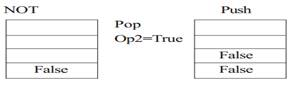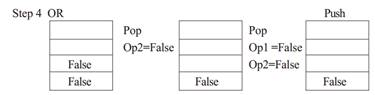Step 5: Push

 True False

Step 6: Push

 False True False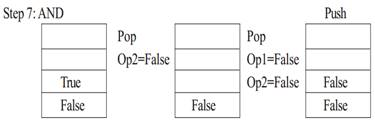Step 8: OR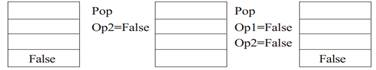Step 9: Pop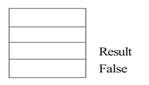OR

Any other method for evaluating the given postfix expression showing the Stack Status.

4. (a) Observe the program segment given below carefully and fill the blanks marked as Statement 1 and Statement 2 using tellg() and seekp() functions for performing the required task. (1)

#include <fstream.h>

class Client

{

long Cno; char Name, Email ;

public:

//Function to allow user to enter the Cno, Name,

Email void Enter() ;

//Function to allow user to enter (modify) Email

void Modify() ;

long ReturnCno() {return Cno;}

};

void ChangeEmail()

{ Client C;

fstream F;

F.open (“INFO.DAT”,ios::binary|ios::in|ios::out);

long Cnoc;//Client’s no. whose Email needs to be

changed cin>>Cnoc;

{

if (Cnoc==C.ReturnCno())

{

C.Modify();

//Statement 1

int Pos = __________//To find the current

position of file pointer

// Statement 2

_______________//To move the file

pointer to write the

//modified record

back onto the file

//for the desired Cnoc

F.write((char*)&C, sizeof(C));

}

}

F.close();

}

Ans. Statement 1:

F. tellg ();

Statement 2:

F. seekp(Pos-sizeof(C)) ;

OR

F.seekp(-sizeof(C), ios::cur);

OR

(b) Write a function in C++ to count the words “this” and “these” present in a text file “ARTICLE.TXT”. (2)

[Note that the words “this” and “these” are complete words]

Ans. void COUNT ( )

{

ifstream Fil;

Fil.open(“ARTICLE.TXT”);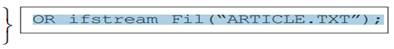char Word 

int C1 = 0, C2 = 0

while (!Fil.eop())

{

Fil>>Word;

if (strcmp(Word,“this”) ==0)

Cl++;

else if (strcmp(Word,“these”) ==0)

C2++;

}

cout<<“Count of -this- in file:”<<Cl;

cout<<“Count of -these- in file:”<<C2;

 OR cout<<“Count of -this- and —these- -in file:”<

Fil.close(); //gnore

}

OR

void COUNT( )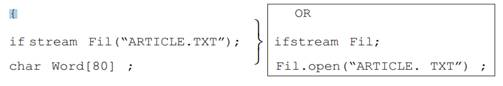int C = 0;

while(!Fil.eof())

{  Fil>>Word;

if (strcmp(Word,“this”)==0||strcmp(Word,“these”) ==0)

C++;

}

cout<<“Count of -this- and -these- in file:”<<C;

Fil.close(); //Ignore

}

OR

void COUNT( )

{

ifstream Fil(“ARTICLE.TXT”);

//OR fstream Fil;

// Fil. open(“ARTICLE. TXT”, ios: : in);

char STR[l0];

int Cl=0, C2=0;

while (Fil.getline (STR,l0, ”))

{

if (strcmp(STR,“this”)==0)

Cl++;

else if (strcmp(STR,“these”)==0)

C2++;

}

}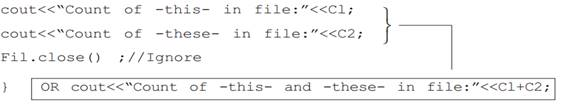OR

void COUNT ( )

{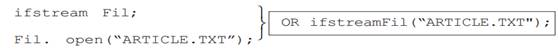Char Word , Ch;
int Cl =0, C2 = 0, I=O;

while(Fil.get(Ch))

{

if (Ch! = ‘ ‘)

Word[I++] = Ch;

else

{

Word[I] = ‘\0’;

if (strcmp (Word,“this”)==0)

Cl++;

else if (strcmp(Word,“these”)==0)

C2++;

I=0,

}

}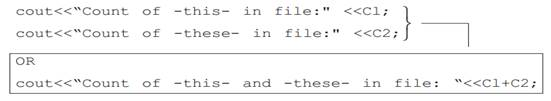Fil.close(); //Ignore

}

OR

Any other correct function definition

(c) Write a function in C++ to search and display details of all flights, whose destination is “Mumbai” from a binary file “FLIGHT. DAT”. Assuming the binary file is containing the objects of the following class. (3)

class FLIGHT

{

int Fno;   //Flight Number

char From; //Flight Starting Point

char To;   //Flight Destination

public:

char* GetFrom() {return From;}

void Enter() {cin>>Fno;gets(From);gets(To);}

void Display(){cout<<Fno<<“:”<<From<<“:”<<To<<endl;}

};

{

FLIGHT F;

ifstream fin;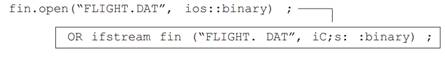{

if (strcmp(F. GetTo(),“Mumbai”))

F.Display() ;

}

fin.close(); //Ignore

}

OR

{

FLIGHT F;

ifstream fin;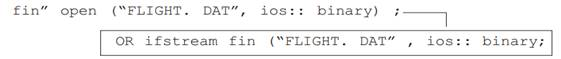if (fin)

{

while(!fin.eof())

{

if (strcmp(F. GetTo(),“Mumbai”))

F.Display();

}

Fin.close(); //Ignore

}

OR

Any other correct function definition

5. (a) What do you understand by Candidate Keys in a table? Give a suitable example of Candidate Keys from a table containing some meaningful data. (2)

Ans. A table may have more than one such attribute/group of attribute that identifies a tuple uniquely, all such attribute(s) are known as Candidate Keys.

Table: Item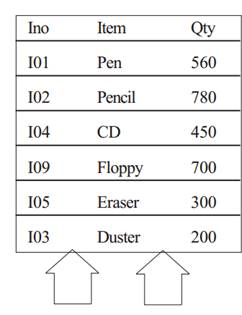(b) Consider the following tables STORE and SUPPLIERS and answer (b1) and (b2) parts of this question:

Table: STORE

 Item No. Item Scode Qty Rate LastBuy 2005 Sharpener Classic 23 60 8 31-June-09 2003 Ball Pen 0.25 22 50 25 01-Feb-10 2002 Gel Pen Premium 21 150 12 24-Feb-10 2006 Gel Pen Classic 21 150 20 11-Mar-09 2001 Eraser Small 22 220 6 19-Jan-09 2004 Eraser Big 22 110 8 02-Dec-09 2009 Ball Pen 0.5 21 180 18 03-Nov-09

Table: SUPPLIERS

 Scode Sname 21 Premium Stationers 23 Soft Plastics 22 Terta Supply

(b1) Write SQL commands for the following statements: (4)

(i) To display details of all the items in the Store table in ascending order of LastBuy.

Ans. SELECT * FROM STORE ORDER BY LastBuy;

(ii) To display ItemNo and Item name of those items from Store table whose Rate is more than 15 Rupees.

Ans. SELECT ItemNo, Item..In FROM STORE WHERE Rate >15;

(iii) To display the details of those items whose Supplier code (Scode) is 22 or Quantity in Store (Qty) is more than 110 from the table Store.

Ans. SELECT * FROM STORE WHERE Scode = 22 OR Qty >110;

(iv) Todisplay Minimum Rate of items for each Supplier individually as per Scode from the table Store.

Ans. SELECT Scode, MIN(Rate) FROM STORE GROUP BY Scode; (b2) Give the output of the following SQL queries: (2)

Note: In all output Questions ignore Column Headings

(i) SELECT COUNT(DISTINCT Scode) FROM Store;

Ans. COUNT(DISTINCT Scode)

(ii) SELECT Rate*Qty FROM Store WHERE ItemNo=2004;

Ans.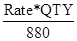(iii) SELECT Item,Sname FROM Store S, Suppliers P WHERE S.Scode=P.Scode AND ItemNo=2006;

Ans. ITEM SNAME

Ans.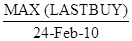These are First 5 questions only. To view and download complete question paper with solution install myCBSEguide App from google play store or login to our student dashboard.

## Last Year Question Paper Class 12 Computer Science 2010

Download class 12 Computer Science question paper with solution from best CBSE App the myCBSEguide. CBSE class 12 Computer Science question paper 2010 in PDF format with solution will help you to understand the latest question paper pattern and marking scheme of the CBSE board examination. You will get to know the difficulty level of the question paper.

## Previous Year Question Paper for class 12 in PDF

CBSE question papers 2018, 2017, 2016, 2015, 2014, 2013, 2012, 2011, 2010, 2009, 2008, 2007, 2006, 2005 and so on for all the subjects are available under this download link. Practicing real question paper certainly helps students to get confidence and improve performance in weak areas.

To download CBSE Question Paper class 12 Accountancy, Chemistry, Physics, History, Political Science, Economics, Geography, Computer Science, Home Science, Accountancy, Business Studies and Home Science; do check myCBSEguide app or website. myCBSEguide provides sample papers with solution, test papers for chapter-wise practice, NCERT solutions, NCERT Exemplar solutions, quick revision notes for ready reference, CBSE guess papers and CBSE important question papers. Sample Paper all are made available through the best app for CBSE students and myCBSEguide website.### Test Generator

Create question paper PDF and online tests with your own name & logo in minutes.### myCBSEguide

Question Bank, Mock Tests, Exam Papers, NCERT Solutions, Sample Papers, Notes